Publicité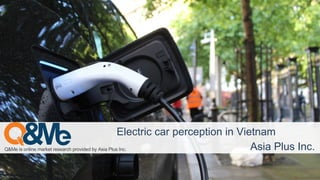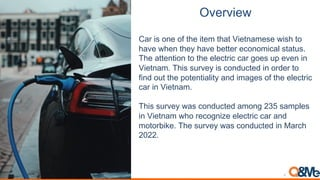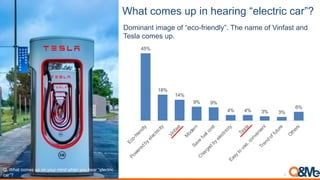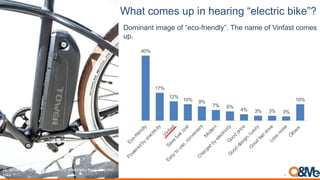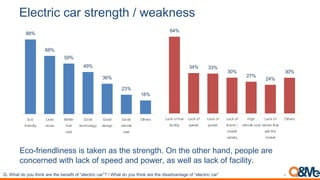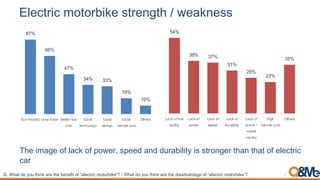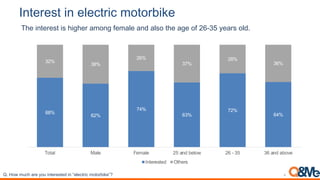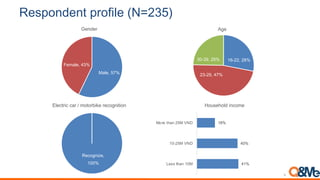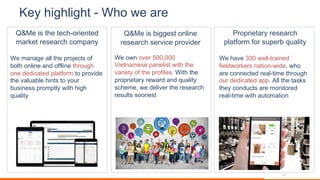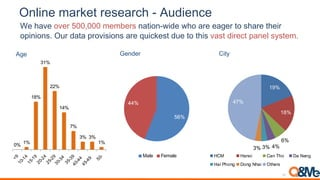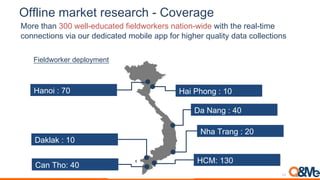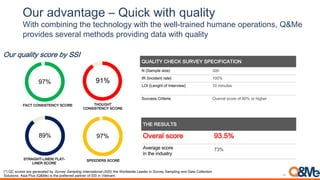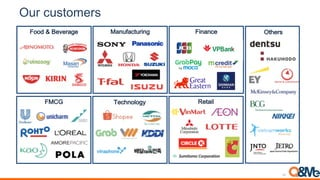1 sur 16
Publicité

### Electric car perception in Vietnam.pdf

1. !"#$%& '()%($ *+,-$. ,$&$+,/0 1,'2%3$3 45 6&%+ 7)8& 9(/: !"#$%&'$($)&(*#&$#*%'+,(',(-'#%,). /0')(1"20(3,$4( 1 2. !"#$"%#& !"#$%&$'()$'*$+,)$%+)-$+,"+$.%)+("-)&)$/%&,$+'$ ,"0)$/,)($+,)1$,"0)$2)++)#$)3'('-%3"4$&+"+5&6$7,)$"++)(+%'($+'$+,)$)4)3+#%3$3"#$8')&$59$)0)( %( .%)+("-6$7,%&$&5#0)1$%&$3'(:53+):$%($'#:)#$+'$*%(:$'5+$+,)$9'+)(+%"4%+1$"(:$%-"8)&$'*$+,)$)4)3+#%3$ 3"#$%($.%)+("-6$7,%&$&5#0)1$/"&$3'(:53+):$"-'(8$;<=$&"-94)&$ %($.%)+("-$/,'$#)3'8(%>)$)4)3+#%3$3"#$"(:$-'+'#2%?)6$7,)$&5#0)1$/"&$3'(:53+):$%($@"#3,$ ;A;;6 2
3. 56)% $+.#0 2*(',(6#)&',7(8#"#$%&')&9: 45% 18% 14% 9% 9% 4% 4% 3% 3% 6% E c o - f r i e n d l y P o w e r e d b y e l e c t r i c i t y V i n f a s t M o d e r n S a v e f u e l c o s t C h a r g e d b y e l e c t r i c i t y T e s l a E a s y t o u s e , c o n v e n i e n t T r e n d o f f u t u r e O t h e r s Dominant image of “eco-friendly”. The name of Vinfast and Tesla comes up. !"#$%&'#()*+,#-.#)/#0)-1#*2/3#4%+/#0)-#%+&1#5+6+('12(# (&178 3 4. 56)%$+.#0 2*(',(6#)&',7(8#"#$%&'$ ;'<#9: 40% 17% 12% 10% 9% 7% 6% 4% 3% 3% 3% 10% E c o - f r i e n d l y P o w e r e d b y e l e c t r i c i t y V i n f a s t S a v e f u e l c o s t E a s y t o u s e , c o n v e n i e n t M o d e r n C h a r g e d b y e l e c t r i c i t y G o o d p r i c e G o o d d e s i g n , l u x u r y G o o d f e e l d r i v e L e s s n o i s e O t h e r s Dominant image of “eco-friendly”. The name of Vinfast comes up. !"#$%&'#()*+,#-.#)/#0)-1#*2/3#4%+/#0)-#%+&1#5+6+('12(# 92:+78 4 5. =+.*),>(?#@#&)%#@(AB(#"#$%&'$($)&(B(;'<# !"#$%&'#()*.&/0#()*+,#-.#4%+/#0)-#%+&1#5+6+('12(#(&17# )1#5+6+('12(#*)')192:+7 Vinfast is dominantly linked with electric car image. Some link Honda / Yamaha with electric image. 5 74% 19% 11% 3% 3% 3% 3% 4% VinFast Tesla Honda Xmen Anbico Yamaha Pega - HK bike Others 6. '(#)*$%)+),$+-*$#./*0+1+&#,2.#-- B3'C*#%)(:4%()&&$%&$+"?)($"&$+,)$&+#)(8+,6$D($+,)$'+,)#$,"(:E$9)'94)$"#)$ 3'(3)#():$/%+,$4"3?$'*$&9)):$"(:$9'/)#E$"&$/)44$"&$4"3?$'*$*"3%4%+16 !"#$%&'#3)#0)-#'%2/:#&1+#'%+#9+/+;2'#);#5+6+('12(#(&178#<#$%&'#3)#0)-#'%2/:#&1+#'%+#32,&3=&/'&>+#);#5+6+('12(#(&17 ;;< =;< >?< @?< A=< BA< C=< !"# $%&'()*+ ,'-- (#&-' .'//'%$0'* "#-/ 1##) /'"2(#*#3+ 1##) )'-&3( 1##) 4'2&"*' "#-/ 5/2'%- 6 =@< A@< AA< AD< BE< B@< AD< ,6"78#$8$0'* $6"&*&/+ ,6"78#$ -9'') ,6"78#$9#:'% ,6"78#$ ;%6()8< =#)'* 46%&'/+ >&32 4'2&"*'8"#-/ ,6"78#$-/#%'-8/26/ -'**8/2' =#)'* 5/2'%- 7. '(#)*$%)+34*4$5%2#+-*$#./*0+1+&#,2.#-- 7,)$%-"8)$'*$4"3?$'*$9'/)#E$&9)):$"(:$:5#"2%4%+1$%&$&+#'(8)#$+,"($+,"+$'*$)4)3+#%3$3"# !"#$%&'#3)#0)-#'%2/:#&1+#'%+#9+/+;2'#);#5+6+('12(#*)')192:+78#<#$%&'#3)#0)-#'%2/:#&1+#'%+#32,&3=&/'&>+#);#5+6+('12(#*)')192:+78 ;E< =;< @E< A@< AA< C?< CD< !"#?$%&'()*+ ,'--8(#&-' .'//'%8$0'* "#-/ 1##) /'"2(#*#3+ 1##) )'-&3( 1##) 4'2&"*'8"#-/ 5/2'%- 7 >@< A;< AE< AC< B=< BA< A>< ,6"78#$8$0'*$6"&*&/+ ,6"78#$9#:'% ,6"78#$ -9'') ,6"78#$)0%6;&*&/+ ,6"78#$ ;%6()8< =#)'* 46%&'/+ >&32 4'2&"*'8"#-/ 5/2'%-
8. 6.*#$#-* %. #(#)*$%) 34*4$5%2# !"#$%&#'#(& $( "$)"#' *+,%) -#+*.#/*%0/*.(, &"#/*)#/,-/12345/6#*'(/,.07 68% 62% 74% 63% 72% 64% 32% 38% 26% 37% 28% 36% Total Male Female 25 and below 26 - 35 36 and above Interested Others 8 !"#?)4#*-(%#&1+#0)-#2/'+1+,'+3#2/#5+6+('12(#*)')192:+78
9. 7#-84.9#.*+8$4:%(#+;<=>?@A @&6+A#BCD E+*&6+A#FGD F$(3$, HIJKKA#KLD KGJKMA#FCD GNJGMA#KBD 6G$ O+()>/2P+A# HNND H)$/.,%/I/+,IJI*'.',4%-$I,$/'G(%.%'( !"# !$# "%# &'(()*+,-)"$. "$/01.)234 .56')*+,-)01.)234 K'8&$0')3I%(/'*$ 9
10. BCD# E F54G*+!.(%.#+D,$2#*+7#-#,$)0+H#$"%)#- 10 11. I#J+0%/0(%/0*+K L04+&#+,$# 89:# $(/&"#/&#;"3,'$#%&#0/ +*'<#&/'#(#*';"/;,+=*%6 !"#$%&%'"#%((#)*"#+,-."/)0#-1# 2-)*#-&(3&"#%&4#-11(3&"#)*,-5'*# -&"#4"43/%)"4#+(%)1-,$#)-#+,-634"# )*"#6%(5%2("#*3&)0#)-#7-5,# 2503&"00#+,-$+)(7#83)*#*3'*# 95%(3)7 89:#$(/>$))#(&/,%.$%#/ '#(#*';"/(#'?$;#/=',?$0#' !"#-8&#-6",#:;;<;;;# =3")&%$"0"#+%&"(30)#83)*#)*"# 6%,3")7#-1#)*"#+,-13("0>#!3)*#)*" +,-+,3")%,7#,"8%,4#%&4#95%(3)7# 0/*"$"<#8"#4"(36",#)*"#,"0"%,/*# ,"05()0#0--&"0) @',='$#&*'6/'#(#*';"/ =.*&-,'+/-,'/(A=#'>/BA*.$&6 !"#*%6"#?;;#8"((@),%3&"4# 13"(48-,A",0#&%)3-&@834"<#8*-# %,"#/-&&"/)"4#,"%(@)3$"#)*,-5'*# -5,#4"43/%)"4#%++>#B((#)*"#)%0A0# )*"7#/-&45/)0#%,"#$-&3)-,"4# ,"%(@)3$"#83)*#%5)-$%)3-& 11
12. !.(%.#+3,$2#*+$#-#,$)0+K FG9%#.)# F)$,"0)$'0)#$=AAEAAA$-)-2)#&$("+%'(C/%:)$/,'$"#)$)"8)#$+'$&,"#)$+,)%#$'9%(%'(&6$D5#$:"+"$9#'0%&%'(&$"#)$G5%3?)&+$:5)$+'$+,%&$0"&+$:%#)3+$9"()4$&1&+)-6 0% 1% 18% 31% 22% 14% 7% 3% 3% 1% Q M H N J H F H B J H M K N J K F K B J K M G N J G F G B J G M F N J F F F B J F M B N J B'" !"# !$# %# &# '# '# &(# ?R@ ?&/)2 R&/#S%) T&#U&/> ?&2#V%)/> T)/>#U%&2 W'%+1, )%# &&# #+)$L$*+)$C"&4", D3)7 12 13. !::(%.#+3,$2#*+$#-#,$)0+K M4"#$,/# @'#)$+,"($<AA$/)44C):53"+):$*%)4:/'#?)#&$("+%'(C/%:)$/%+,$+,)$#)"4C+%-)$ 3'(()3+%'(&$0%"$'5#$:):%3"+):$-'2%4)$"99$*'#$,%8,)#$G5"4%+1$:"+"$3'44)3+%'(&$$H!@IJ<A !"(7,'IKA L"M"(8IKA$$ H"('%$I$NA$M," 7#"(8$I$;A$ H"%$O,'(8 I$JA$L"?4"? I$JA$Fieldworker deployment 13 14. !G$ ,9",.*,/# E BG%)2 &%*0 NG,(%*J F%+,$3'-2%(%(8$+,)$+)3,('4'81$/%+,$+,)$/)44C+#"%():$,5-"()$'9)#"+%'(&E$PQ@) 9#'0%:)& &)0)#"4 -)+,':& 9#'0%:%(8 :"+" /%+, G5"4%+1 CA'/BA*.$&6/(;,'#/>6/DDE @ABC8B5DEFEC!DBG8EB5H! 97% C>5I1>C8 B5DEFEC!DBG8EB5H! 91% ECHAF1>C?,FD!H<8@,AC? ,FD!H8EB5H! 89% EJ!!K!HE8EB5H! 97% !M6N9OP QKHQRISMTUHPIS7HQ9L9Q6O9VW 3 78,9:;')(<='> ?$$@A)7@-B<C'-*)6,*'> "$$# &D@)7&'-E+*)5F)@-*'6G<'H> "$)9<-I*'( 8IBB'(()J6<*'6<, DG'66,;)(B56')5F)%$# 56)+<E+'6) OKH THSMNOS C?#'*./(;,'# F475G 62$,+G$I&/',$%(I.0$I%(38&.,5 EA< LMN8OB8-"#%'-86%'83'('%6/')8;+8E0%4'+8E6=9*&(38F(/'%(6/&#(6*8LEEFNP8/2'8Q#%*):&)'8,'6)'%8&(8E0%4'+ E6=9*&(3 6()8K6/68B#**'"/&#(8 E#*0/&#(-R8A-&68J*0-8LOST'N8&-8/2'89%'$'%%')896%/('%8#$8EEF8&(8U&'/(6=R8 14
15. !G$)G-*43#$- E--4#F#G"6",%'" EHDC H%&51%/)5,3&' I"/*&-(-'7 E3&%&/" J)*",0 K")%3( 15
16. Q&Me is provided by Asia Plus Inc. https://qandme.net Contact us: Tel: 02839 100 043 Email: info@qandme.net 16
Publicité